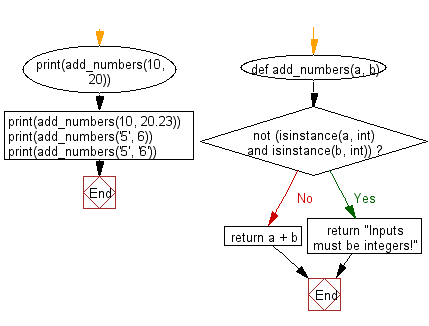﻿ Python: Add two objects if both objects are an integer type - w3resource# Python: Add two objects if both objects are an integer type

## Python Basic: Exercise-36 with Solution

Write a Python program to add two objects if both objects are an integer type.

Sample Solution:

Python Code:

``````def add_numbers(a, b):
if not (isinstance(a, int) and isinstance(b, int)):
return "Inputs must be integers!"
return a + b
```
```

Sample Output:

```30
Inputs must be integers!
Inputs must be integers!
Inputs must be integers!
```

Flowchart:## Visualize Python code execution:

The following tool visualize what the computer is doing step-by-step as it executes the said program:

Python Code Editor:

Have another way to solve this solution? Contribute your code (and comments) through Disqus.

What is the difficulty level of this exercise?

Test your Python skills with w3resource's quiz

﻿

## Python: Tips of the Day

Unpack variables from iterable:

```# One can unpack all iterables (tuples, list etc)
>>> a, b, c = 1, 2, 3
>>> a, b, c
(1, 2, 3)

>>> a, b, c = [1, 2, 3]
>>> a, b, c
(1, 2, 3)
```